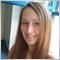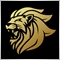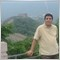# Need help with Last Trade Open Price.1031

This is my function for the last trade opening price.

{

double result = 0;

for(int i = OrdersTotal()-1; i >= 0; i--)

{

if(OrderSymbol() == Symbol() && OrderMagicNumber() == MagicNumber && (OrderType() == OP_BUY || OrderType() == OP_SELL))

{

result = OrderOpenPrice();

break;

}

}

return(result);

}

Now I would like to compare it to the current price. I wrote this.

Bid > LastTradePrice() +/- 100 * myPoint //Price > Last Open Trade Price + fixed value

How would I make sure this is a positive number always as the last trade could have opened on either side of the bid. I just need to make sure that number will always be positive.

Thank you.732

You can check after that if the value is positive and only if positive go on with your code1031

So is this correct? Will this work whether last open trade price was above or below the current bid? This will return a positive number?

MathAbs( Bid - LastTradePrice() ) < 50 * myPoint

Thanks.732

It returns always a value >= 0

So if your value is -5.21 it returns 5.21, if your value is 5.21 it returns 5.211031

Thanks. So will the above format work as I want it to?

Thanks.732

I don't know what you want ;)Moderator
123911544

It seems to me you are doing Grid or Martingale code ...

In your case, you must know that whenever price above last opened order by a certain number of pips (PipStep) , you have to open Sell order

and when price is down the last opened trade with a certain number of pips you have to open Buy order ... right?

Then, if you used the proper formula, you will never get negative numbers ...

Please check my own code I used to use ...

```//In the start() function ...
NewLots = NormalizeDouble(LastLotsUP(Symbol())*LotExponent,LotDigits);
if(NewLots == LastLotsUP(Symbol())) NewLots = NormalizeDouble((NewLots+Lots),LotDigits);
}

NewLots = NormalizeDouble(LastLotsDN(Symbol())*LotExponent,LotDigits);
if(NewLots == LastLotsDN(Symbol())) NewLots = NormalizeDouble((NewLots+Lots),LotDigits);
if(AllowSellTrades) {OpenSell(NewLots, MagicDN, TakeProfit, StopLoss, Expert_Name); return(0);}
}
}

...
...
...
// Out of the start() function ...

int cnt, total;
total = OrdersTotal();
double oprice=0;

for(cnt=0; cnt<total; cnt++){

oprice = OrderOpenPrice();
}
}
return(oprice);
}

double LastOpenSellPrice(string smbl){
int cnt, total;
total = OrdersTotal();
double oprice=0;

for(cnt=0; cnt<total; cnt++){

if(OrderSymbol()==smbl && OrderType()==OP_SELL && OrderMagicNumber()==MagicDN){
oprice = OrderOpenPrice();
}
}
return(oprice);
}
```

Note that I use separate functions for both Buy and Sell orders logic.

This makes things easier, I guess ...1031

Thanks. I am spacing trades out but not a grid strategy.